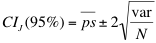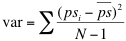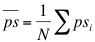Comparing Partitions
Getting started
Diversity
Pairwise agreement
Information theory
Calculations

## Approximated 95% Confidence Intervals calculation by jackknife

Since the measured congruence is dependent on the particular sample taken from the population, there is variability in the estimated values relatively to those of the true population (Pinto et al., 2008). The use and interpretation of the congruence measures can be improved by estimation of suitable confidence intervals (CIs). However, for most of the congruence measures, there is no analytical formula for CIs calculation.

To better understand how statistically significant is the data set under analysis, and how the sampling can affect results, resampling techniques can be used to estimate CIs. We recently compared the use of different resampling techniques for CI calculation. Our results shown the CIs estimated by jackknife pseudo-values matching, in some cases, and outperforming, in other cases, the CIs calculated by bootstrap (Severiano et al, 2011). Therefore, we recommend the use of jackknife pseudo-values CI when an analytical formula is not available. To calculate the jackknife pseudo-values confidence interval, click in "95% CI estimation by resampling" bellow the results' table.

• ### 9.1. The jackknife pseudo-values approach

The delete-one jackknife relies on resamples that leave out one entity of the sample at a time, where entities are those individuals that are randomly sampled from the population. A pseudo-values approach was used to calculate the jackknife CIs. For an estimator S, the ith pseudo-value of S was calculated as psi=NS-(N-1)Si where Si is the estimator value for the sample with the ith data point deleted. The jackknife CI was then calculated aswhereand• ### 9.2. Previous versions

Two bootstrap methods were initially made available for the 95% confidence interval estimation: Percentile method and Bias Corrected and accelerated method (BCa). In this later version of the website, we no longer present the confidence intervals calculation by this bootstrap methods. According to our results, the jackknife pseudo-values method outperforms both Bootstrap methods, with faster computation times. Although we recomend the use of jackknife pseudo-values for the CI estimation, you can use this link to calculate bootstrap BCa and percentile CIs to confirm previous results.

You can also expand this text to see further information about the display of bootstrap distributions.